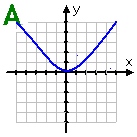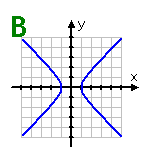Symmetry
Examples

For each of the following graphs, list any symmetry. Then state if the graph represents an even or odd function.Graph A: This graph is symmetric about the line x = 0 (the y-axis). There is no other symmetry. It is considered an even function.Graph B: This graph is symmetric about the lines x = 0 (the y-axis) and y = 0 (the x-axis), and also about the origin. However, since there exists vertical lines (such as the line x = 2) which will cross this graph twice, it does not represent a function.

Listen